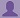SHARE
HELP

To start with, Sr2Jr’s first step is to reduce the expenses related to education. To achieve this goal Sr2Jr organized the textbook’s question and answers. Sr2Jr is community based and need your support to fill the question and answers. The question and answers posted will be available free of cost to all.# Computer Networking : A Top-down Approach

Authors:
James F. Kurose, Keith W. Ross
Exercise:
Problems
Chapter:
Transport Layer
Edition:
6
ISBN:
9780132856201
Question:
52
 Previous Next

###Question

Consider a modification to TCP’s congestion control algorithm. Instead of additive increase, we can use multiplicative increase. A TCP sender increases its window size by a small positive constant a (0 < a < 1) whenever it receives a valid ACK. Find the functional relationship between loss rate L and maximum congestion window W. Argue that for this modified TCP, regardless of TCP’s average throughput, a TCP connection always spends the same amount of time to increase its congestion window size from W/2 to W.

###Answer

The maximum window size is represented by w.

The total number of segments(S) sent out during the interval when TCP changes its window size from w/2 up to and includes w.

S=w/2 +(w/2)*(1+a)+(w/2)*(1+a)^2 +(w/2)*(1+a)^3 +(w/2)*(1+a)^4 + . . .+ (w/2)*(1+a)^n

Here,n=log(1+a) 2,then S=w*(2a+1)/(2a).

The Loss rate is derived by

L=1/S= (2a)/ (w*(2a+1)).

The TCP takes time to increase its window size from w/2 to w is calculated by

n*RTT = (log(1+a) 2 * RTT

This is independent of TCP’s average throughput.

TCP’s average throughput is derived by

Throughput B=MSS*S/((n+1)*RTT) =MSS/(L*(k+1)*RTT)

Note that the derived throughput is different from original throughput.

### Discussions

Post the discussion to improve the above solution.